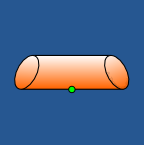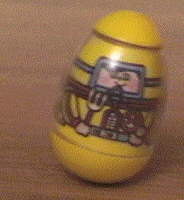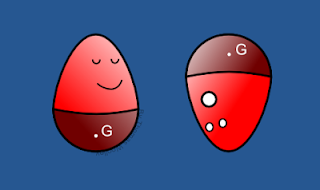## Monday, 21 January 2013

### Lazy eggsFigure 1. Lazy eggs don't stand up.
Last week I introduced the idea of stable and unstable equilibrium points using weebles as an example. As I mentioned, they work because their bottom is much heavier than the rest of the toy. However, suppose now that the weeble is constructed from a completely uniform material. Thus, the bottom would only be heavier if it had a larger volume of substance was put there. Would the weeble still work? This is the question we will be starting to look at this week.

It may surprise you but the simple answer is actually in your fridge! An uncooked egg’s density is pretty constant throughout its shape. There are small differences between the shell, albumen and yolk but they are unimportant and it is obvious to see that eggs don’t stand to attention . The eggs actually have an infinite number of equilibrium points because they can be rolled over to any point on their side and they won’t move.

So a uniform egg shape won’t stand up, but will any shape? Mathematically, what we are looking for is a shape that has exactly one stable equilibrium point and one unstable equilibrium point.

Before we consider the 3D case, let us remove a dimension and consider flat 2D shapes. For two-dimensional convex shapes it can be proven that:

all planar, convex, homogenous shapes have at least 2 stable and 2 unstable equilibria.

A sketch of the proof can be found below. Hence, we know that if a weeble of uniform density exists then it cannot be effectively two-dimensional. What we can observe is that the 2D oval has two stable equilibria and for any number greater or equal to three the regular polygon with “n” sides will have “n” stable equilibria.

But what about three dimensions? Sadly, things are not so simple. However, when logical proofs are not forthcoming mathematicians are just as open to experimentation as any other scientist.

Below are a number 3D shapes, have a go at counting the number of stable equilibria they have. The answers can be found if you scroll down past the theorem proof.Figure 2. A cube, a square based pyramid and a cylinder with edges sliced at an angle.
Now that you have got a feel for finding stable equilibria, have a think about what kind of shape would have one stable equilibrium point and one unstable only. Are you sure it even exists? Before we answer this question we take a detour next week and show how we can make our eggs not so lazy!

________________________________________________________________
________________________________________

 It is possible to get your eggs to stand up right if you hard boil them and give them a spin. http://charlottemasonway.blogspot.co.uk/2012/09/weekly-wrap-up-week-4.html

________________________________________________________________
________________________________________

Proof of theorem 1

Consider a 2D convex object that has only one stable and one unstable equilibrium point. Define a function, R(ɵ), that is the distance of the perimeter away from the centre of gravity as shown in the figure on the left. The function depends on the angle ɵ (measured in radians) around the centre of gravity and so as ɵ increases from 0 to 2π the function, R, will do one revolution of the shape.

Now consider the graph of R. By assumption there are only two equilibria, one stable and one unstable, thus, the corresponding graph has just one maximum and one minimum, as shown in the figure on the right. This is how the equilibria where defined last week.

Suppose we now drop a horizontal line across the graph. In particular let the points at which the horizontal line touches the graph be separated by an angular distance of π radians. This will correspond to a straight cut through the shape. Call this corresponding value R0.

By definition everything above this horizontal would be the part of the shape that is further than R0 away from the centre of gravity and everything below would the part of the shape closer than R0 to the centre of gravity. This means that one half of the shape would be bigger than the other half, and, thus, heavier. But by definition the cut goes through the centre of gravity and so each side weighs the same. As we come to a contradiction, our original hypothesis (that such a shape exists) must be wrong.
________________________________________________________________
________________________________________The cube has 6 stable equilibria; one on each of the flat faces, as shown.The cube has 5 stable equilibria; one on each of the flat faces, as shown.The sliced cylinder only has 1 stable equilibrium. However, it is not a shape that satisfies our need because it has 3 unstable equilibria; one on the top of the cylinder and one at each of the tips.

## Monday, 7 January 2013

### Sense and instabilityFigure 1. A worried little weeble.
Did you ever have set of weebles? I did. They were wonderful little toys that lived up to their advertising slogan of “weebles wobble, but they don’t fall down”. The whole premise was that you got a set of several small plastic egg shaped characters (as seen in Figure 1) that would always wobble back to an upright position, no matter what position you started them from. As you can imagine hours of fun could be had with these little things.

The toy's mechanism is very simple. The bottom of the weeble is much heavier than the rest of the body, this means its centre of gravity is very low. Due its egg shaped body the weeble will always wobble such that its centre of gravity is at its lowest point. The applet below shows how this idea works . On the left shape click on the red star which is the centre of mass and move it around the shape. The effect can be seen in the shape on the right. The shape on the right can then be grabbed and started from different initial points. However, it will always evolve to a stable point.

Why am I talking about weebles? Well, they are a really nice way of demonstrating how mathematicians understand equilibrium points, stability and instability. Although theoretically if a body was placed at its equilibrium points nothing further would occur, we know that physically we cannot be that accurate. Thus, equilibrium points can then be separated (generally) into stable and unstable points . Thankfully, the mathematical notion of stability accords with our everyday use of the word. A body is stable if given a small push (or perturbation) it returns back to its previous position.Figure 2. A wobbling weeble.
For a concrete example of this consider Figure 3, which is a schematic diagram of a curved bowl containing some balls. A is a stable equilibrium point; if the ball is given a small push it will return back to A. If the ball is given a big enough push it can roll right over the dividing line into the C position and this is also a stable equilibrium point. Now consider placing a ball at B. Since the bowl is completely flat there B is also an equilibrium point. However, it is an unstable equilibrium point. This is because no matter how small a push the ball is given it will never head back to B, but rather roll to one of A or C. Importantly, stability is only defined at points A, B and C because these are the only equilibrium points.Figure 3. Illustrating stable and unstable equilibrium points.
A similar idea is applicable to the states of the weeble. When the weeble is upright it is equivalent to the ball being at A or C; the weeble is at a stable equilibrium point. Now suppose you could balance the weeble perfectly on its head, such that the centre of gravity, G, is directly above the flat point of its head, as in Figure 4. This is equivalent to the point B in Figure 2. It is an equilibrium point and, so, theoretically, if placed there, with no perturbation, then the weeble would stay there forever. However, any perturbation, no matter how small, will cause it to flip over and turn the right way up.Figure 4. Stable and unstable weebles.
In two weeks time I will continue this topic and see what happens when we remove the possibility of weighting the bottom and only depend on the geometry of the object.
________________________________________________________________
________________________________________

 All credit of the applet goes to this website: http://l.d.v.dujardin.pagesperso-orange.fr/ct/cusp.html

 Note that there are also saddle points and centres but we’re not going to talk about them as they’re special cases that few people care about. Also, the stable and unstable points could each be further split up into oscillatory and non-oscillatory points but again we’re going to ignore this complexity.